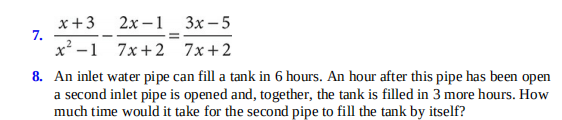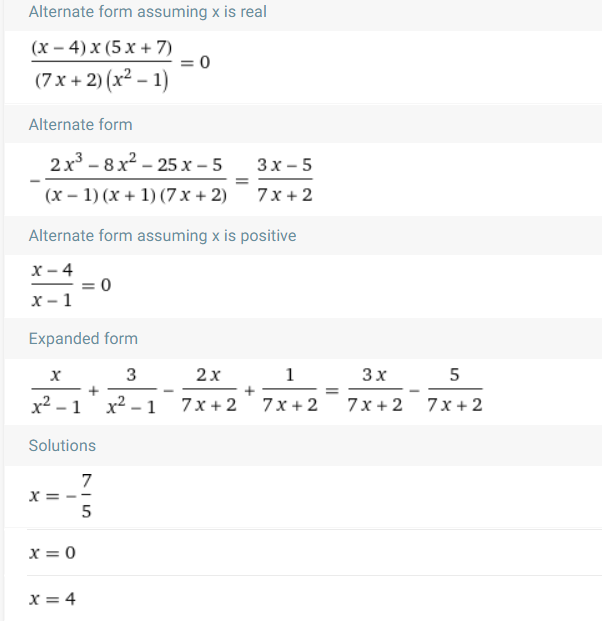### ¿Todavía tienes preguntas de matemáticas?

Pregunte a nuestros tutores expertos
Algebra
Pregunta7. $$\frac { x + 3 } { x ^ { 2 } - 1 } - \frac { 2 x - 1 } { 7 x + 2 } = \frac { 3 x - 5 } { 7 x + 2 }$$

8. An inlet water pipe can fill a tank in $$6$$ hours. An hour after this pipe has been open a second inlet pipe is opened and, together, the tank is filled in $$3$$ more hours. How much time would it take for the second pipe to fill the tank by itself?